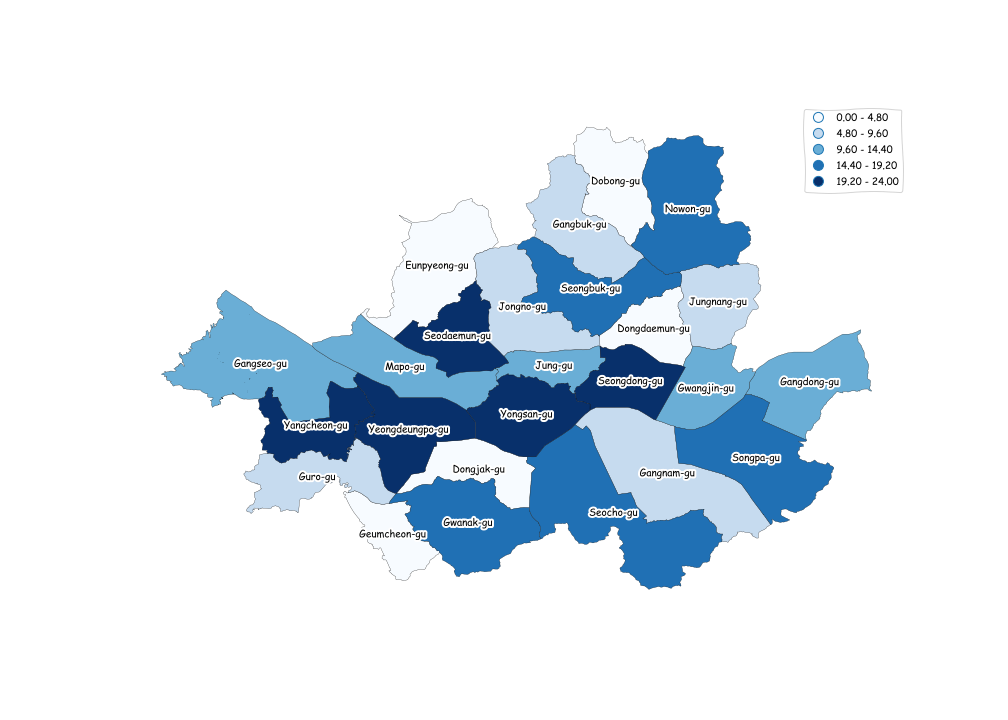# How to define boundary numbers without decimal points - Geopandas

Problem
The numbers in a legend has the decimal points (eg. 0.00-4.80 at the top of the legend box), when I want them to be integers without decimal points. All I care is that the boundary values are integers. It doesn't matter whether a certain region in a choropleth is labelled as the category A or B, as long as the A, B and other categories are separated by the integer values so that legend box shows only integer separations.

It's because I am making these maps for publishing articles after the Korean election is over. It would be much easier for the newspaper readers to have the integer numbers in legend!

My current graphMy code

``````import geopandas as gpd
import matplotlib.pyplot as plt

plt.xkcd()
# https://github.com/southkorea/seoul-maps/tree/master/juso/2015/json

data_result.plot(figsize=(14,10),linewidth=0.25, edgecolor='black', column='ESRI_PK',cmap='Blues',scheme='quantiles',legend=True)

for index,row in seoul.iterrows():
xy=row['geometry'].centroid.coords[:]
xytext=row['geometry'].centroid.coords[:]
plt.annotate(row['SIG_ENG_NM'],xy=xy, xytext=xytext,  horizontalalignment='center',verticalalignment='center')
plt.axis('off')

plt.show()
``````
• What do you mean by 'boundary'? – Kadir Şahbaz May 5 '18 at 9:58
• @Kadir Sorry for not clarifying the meaning of 'boundary'! Boundary here means each of 5 classifications that are in legends. To be precise, what I meant by boundaries are '0.00 - 4.80' , '4.80 - 9.60', '9.60 - 14.40', '14.40 - 19.20' and '19.20 - 24.00'. And my question is whether I can make those classification done with integers, not with float (0.00, 4.80, etc) – Eiffelbear May 5 '18 at 11:27

One way to add integer values to the label is by making the legend yourself with `plt.legend()` and adding Patches to this legend with the correct labels and colours.

``````import geopandas as gpd
import matplotlib.cm as cm
import matplotlib.pyplot as plt
import matplotlib.patches as mpatches

plt.xkcd()
# https://github.com/southkorea/seoul-maps/tree/master/juso/2015/json

seoul.plot(figsize=(14,10),linewidth=0.25, edgecolor='black', column='ESRI_PK', cmap='Blues', scheme='quantiles')

for index, row in seoul.iterrows():
xy = row['geometry'].centroid.coords[:]
xytext = row['geometry'].centroid.coords[:]
plt.annotate(row['SIG_ENG_NM'], xy=xy, xytext=xytext, horizontalalignment='center', verticalalignment='center')
plt.axis('off')

cmap = cm.get_cmap('Blues')
white_patch = mpatches.Patch(color=cmap(0.0), label='0 - 5')
lowblue_patch = mpatches.Patch(color=cmap(0.25), label='5 - 10')
midblue_patch = mpatches.Patch(color=cmap(0.5), label='10 - 14')
highblue_patch = mpatches.Patch(color=cmap(0.75), label='14 - 19')
veryblue_patch = mpatches.Patch(color=cmap(1.0), label='19 - 24')
plt.legend(handles=[white_patch, lowblue_patch, midblue_patch, highblue_patch, veryblue_patch])

plt.show()
``````